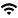# IQUIST Master Calendar

View Full Calendar

## Pingan Cheng "Lower Bounds for Semialgebraic Range Reporting"

Event Type
Seminar/Symposium
Illinois Computer Science
Location
3401 Siebel Center for Computer Science
VirtualDate
Apr 24, 2023   8:30 am
Speaker
Pingan Cheng (Aarhus)
Contact
Candice Steidinger
E-Mail
steidin2@illinois.edu
Views
26
Originating Calendar
Computer Science Speakers Calendar

We look forward to seeing you in-person in 3401 SC or online on Monday, 4/24.

Abstract:

In the semialgebraic range searching problem, we are to preprocess n points in ℝ^d s.t. for any query range from a family of constant complexity semialgebraic sets, all the points intersecting the range can be reported or counted efficiently. When the ranges are composed of simplices, the problem can be solved using S(n) space and Q(n) query time with S(n)Q(n)^d=Õ(n^d) and this trade-off is almost tight. Consequently, there exist low space structures that use Õ(n) space with O(n^{1−1/d}) query time and fast query structures that use O(n^d) space with O(log n) query time. However, for the general semialgebraic ranges, only low space solutions are known, but the best solutions match the same trade-off curve as the simplex queries. It has been conjectured that the same could be done for the fast query  case but this open problem has stayed unresolved.  Here, we disprove this conjecture. We give the first nontrivial and almost tight lower bound for semilagebraic range reporting. In this talk, we study the problem of reporting the points between two polynomials of form Y = ∑_{i=0}^Δ a_i X^i where a_0, ⋯, a_Δ are given at the query time. We show a lower bound of S(n)=Ω(n^{Δ+1}−o(1)/Q(n)^{Δ(Δ+3)/2}). So for the fast query case, i.e., Q(n) = O(log^O(1) n), we get a space lower bound of Ω(n^{Δ+1−o(1)}). This refutes the conjecture that semialgebraic range reporting can be solved as efficiently as its simplex counterpart in the fast query case, and the lower bound actually almost matches the recent upper bound by Agarwal et al. [AAEZ’19].

Based on joint work with Peyman Afshani.  SoCG '21.  https://arxiv.org/abs/2012.00704 .